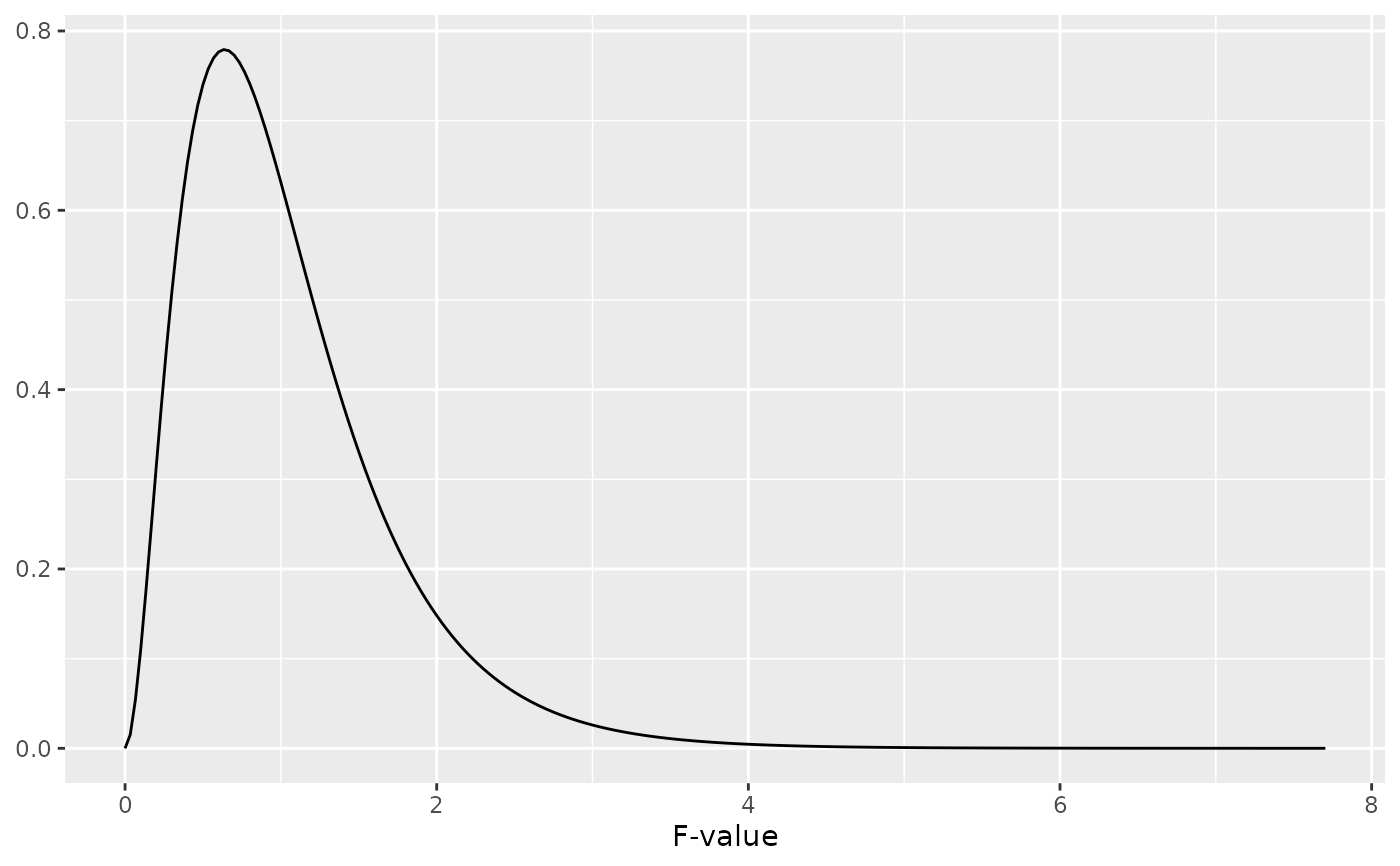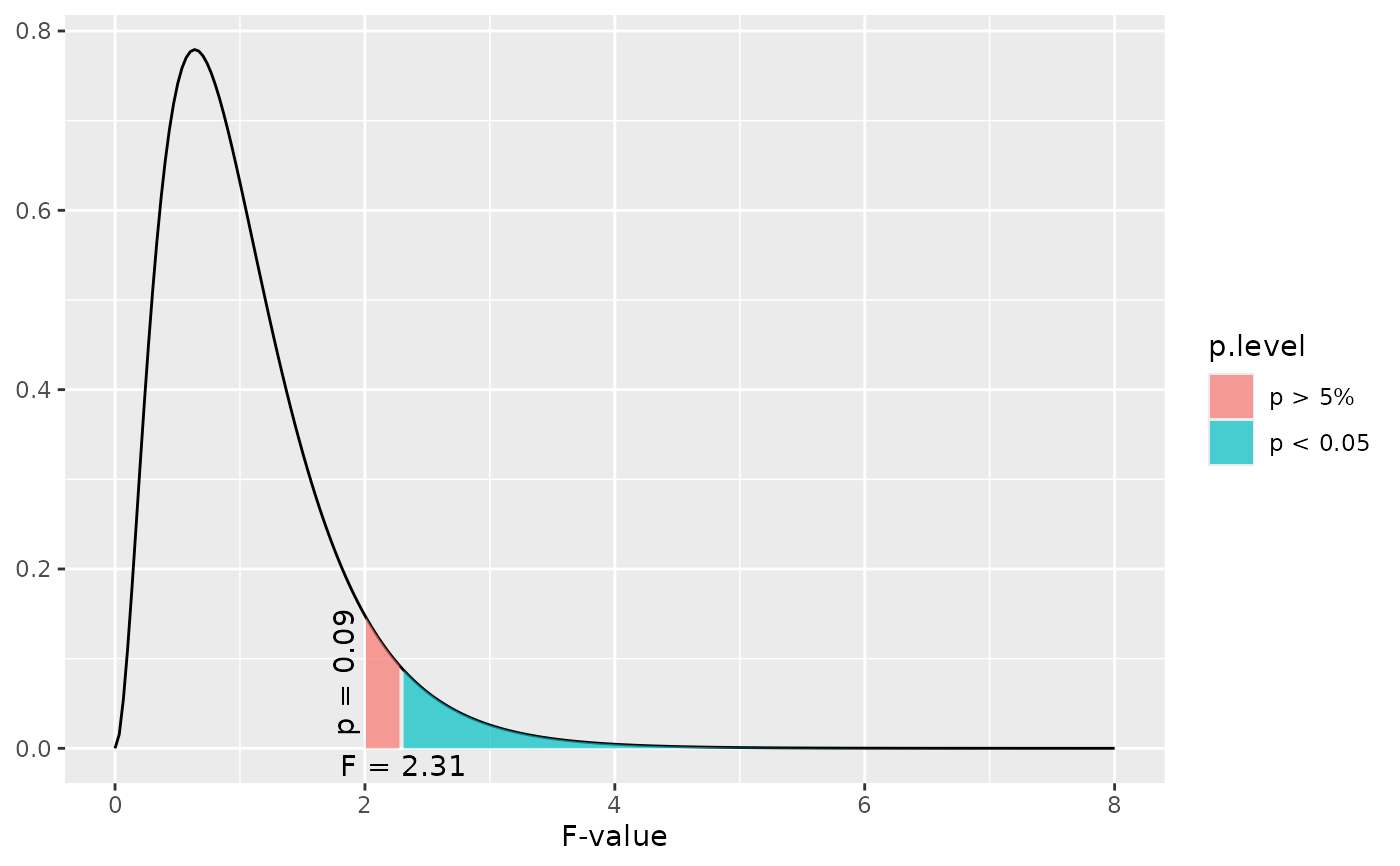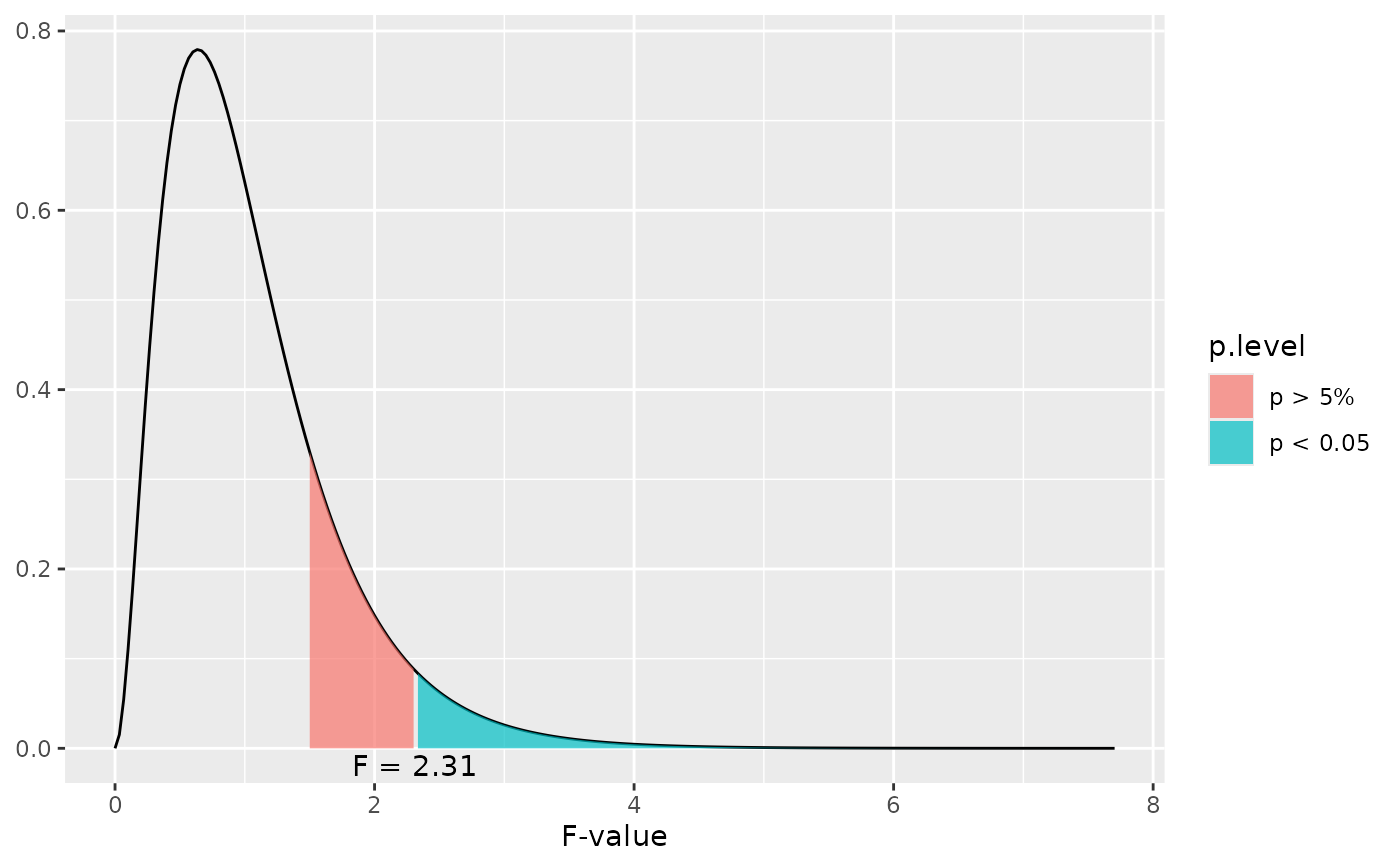This function plots a simple F distribution or an F distribution with shaded areas that indicate at which F value a significant p-level is reached.

dist_f(
f = NULL,
deg.f1 = NULL,
deg.f2 = NULL,
p = NULL,
xmax = NULL,
geom.colors = NULL,
geom.alpha = 0.7
)

Arguments

f

Numeric, optional. If specified, an F distribution with deg.f1 and deg.f2 degrees of freedom is plotted and a shaded area at f value position is plotted that indicates whether or not the specified value is significant or not. If both f and p are not specified, a distribution without shaded area is plotted.

deg.f1

Numeric. The first degrees of freedom for the F distribution. Needs to be specified.

deg.f2

Numeric. The second degrees of freedom for the F distribution. Needs to be specified.

p

Numeric, optional. If specified, a F distribution with deg.f1 and deg.f2 degrees of freedom is plotted and a shaded area at the position where the specified p-level starts is plotted. If both f and p are not specified, a distribution without shaded area is plotted.

xmax

Numeric, optional. Specifies the maximum x-axis-value. If not specified, the x-axis ranges to a value where a p-level of 0.00001 is reached.

geom.colors

user defined color for geoms. See 'Details' in plot_grpfrq.

geom.alpha

Specifies the alpha-level of the shaded area. Default is 0.7, range between 0 to 1.

Examples

# a simple F distribution for 6 and 45 degrees of freedom
dist_f(deg.f1 = 6, deg.f2 = 45)# F distribution for 6 and 45 degrees of freedom,
# and a shaded area starting at F value of two.
# F-values equal or greater than 2.31 are "significant"
dist_f(f = 2, deg.f1 = 6, deg.f2 = 45)# F distribution for 6 and 45 degrees of freedom,
# and a shaded area starting at a p-level of 0.2